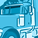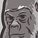# App Development

Announcements
April 22, 2PM EST: Learn about GeoOperations in Qlik Sense SaaS READ MORE
cancel
Showing results for
Did you mean:Creator II

## Invalid dimension using expression

I would like to create a Dimension field using expression but I get invaldi dimension

My expression is

=if([TRADE DATE (P0561)]>min([ACCTG DATE (P0045)]), 'new',
if([MATURITY DATE (P0288)]<max([ACCTG DATE (P0045)]),'matured',
if([MATURITY DATE (P0288)]>max([ACCTG DATE (P0045)]) and [Current\$]=0 and [Last\$]>0,'terminated',
'existed')))

Below is my expected result.  Hope I can get some guidance.  Many thanks.

 status GLOBAL TRADE NUM (P0221) TRADE DATE (P0561) MATURITY DATE (P0288) ACCTG DATE (P0045) Current\$ Last\$ Exist 1000328H 20120419 20200423 20201130 20 30 New 1000328H 20120119 20200423 20201130 10 0 Matured 1001853H 20120423 20190425 20191231 50 Terminated 1001853H 20120423 20200425 20191231 20
Labels (1)
• ### Qlik Sense

1 Solution

Accepted SolutionsCreator II
Author

Thank you very much Sunsun566 and Maylivahanan

I have tried the advise from Maylivahanan and it works.  Thank you very much all for your expertise.

8 RepliesMVP

@43918084 , Try putting 'Total' identifier in all min/max functions in your calculated dimension.Creator II
Author

Thank you very much Tresesco.  I apologise that I don't quite get what you mean.  Would you mind to give me an example?MVP

@43918084 , Try this:

=if([TRADE DATE (P0561)]>min(Total [ACCTG DATE (P0045)]), 'new',
if([MATURITY DATE (P0288)]<max( Total [ACCTG DATE (P0045)]),'matured',
if([MATURITY DATE (P0288)]>max( Total [ACCTG DATE (P0045)]) and [Current\$]=0 and [Last\$]>0,'terminated',
'existed')))MVP

In dimension, you can't use aggregation function like Min(), sum(), it will display invalid dimension.

To avoid this, you need to aggr() function, like

Aggr(if(urcondition), yourdimensions)

Hope it helps

Thanks & Regards,
Mayil Vahanan RContributor III

Hi

Hope it helps you 🙂 from my Stock Aging

IF(AGGR(Max([Transaction date]) - Max([Manufacturing date]),[Lot number]) >= 721 , '24M Up' ,
IF(AGGR(Max([Transaction date]) - Max([Manufacturing date]),[Lot number]) >= 541 , '18-24M' ,
IF(AGGR(Max([Transaction date]) - Max([Manufacturing date]),[Lot number]) >= 451 , '15-18M' ,
IF(AGGR(Max([Transaction date]) - Max([Manufacturing date]),[Lot number]) >= 361 , '12-15M' ,
IF(AGGR(Max([Transaction date]) - Max([Manufacturing date]),[Lot number]) >= 181 , '06-12M' ,
IF(AGGR(Max([Transaction date]) - Max([Manufacturing date]),[Lot number]) >= 151 , '05-06M' ,
IF(AGGR(Max([Transaction date]) - Max([Manufacturing date]),[Lot number]) >= 121 , '04-05M' ,
IF(AGGR(Max([Transaction date]) - Max([Manufacturing date]),[Lot number]) >= 91 , '03-04M' ,
IF(AGGR(Max([Transaction date]) - Max([Manufacturing date]),[Lot number]) >= 61 , '02-03M' ,
IF(AGGR(Max([Transaction date]) - Max([Manufacturing date]),[Lot number]) >= 31 , '01-02M' ,
IF(AGGR(Max([Transaction date]) - Max([Manufacturing date]),[Lot number]) >= 0 , '< 01M' , 'Other'
)))))))))))Creator II
Author

Thank you very much Tresesco and MayilVahanan.

I have tried both and the Agg() advice from MayilVahanan returns no error.

=Aggr(if([TRADE DATE (P0561)]>min([ACCTG DATE (P0045)]), 'new', if([MATURITY DATE (P0288)]<max([ACCTG DATE (P0045)]),'matured', if([MATURITY DATE (P0288)]>max([ACCTG DATE (P0045)]),'terminated', 'existed'))),'status')

But it does not give me any Value, it only returns '-'.  Just wondering if I have done something wrong?MVP

Here 'status' is not the dimension, its string. May be try like below

=Aggr(if([TRADE DATE (P0561)]>min([ACCTG DATE (P0045)]), 'new', if([MATURITY DATE (P0288)]<max([ACCTG DATE (P0045)]),'matured', if([MATURITY DATE (P0288)]>max([ACCTG DATE (P0045)]),'terminated', 'existed'))),[GLOBAL TRADE NUM (P0221)])

As suggested by @tresesco , you can include Total also.  its upto ur requirement.

Thanks & Regards,
Mayil Vahanan RCreator II
Author

Thank you very much Sunsun566 and Maylivahanan

I have tried the advise from Maylivahanan and it works.  Thank you very much all for your expertise.